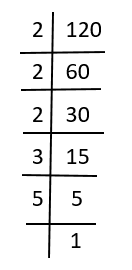# What is Prime Factorisation?

Prime factorisation is a method to find the prime factors of a given number, say a composite number. These factors are nothing but the prime numbers. A prime number is a number which has only two factors, i.e. 1 and the number itself.

For example, 2 is a prime number which has two factors, 2 × 1. Whereas a composite number has more than two factors present in it. For example, 4 has three factors such as 1 × 2 × 2.

## What are Factors and Prime Factors?

Factors: The numbers which are multiplied to get another number. For example, 3 and 5 are the factors of 15, i.e. 3 × 5 = 15.

Prime Factors: A factor which is a prime number and not a composite number is a prime factor. For example, 2, 3 and 5 are the prime factors of 30.

### List of Prime Numbers

The list of prime factors from 1 to 100 are;

 2, 3, 5, 7, 11, 13, 17, 19, 23, 29, 31, 37, 41, 43, 47, 53, 59, 61, 67, 71, 73, 79, 83, 89, and 97

### How to Find Prime Factors?

We can find prime factors with the help of the prime factorisation method. Suppose we need to find the prime factors of a given number, then we have to divide that number by the least prime number, leaving no remainder. Again divide the produced quotient with the least prime number and repeat the steps until the quotient is produced as 1. Let us solve some problems based on prime factorisation to understand the steps better.

## Solved Examples

Q.1: What is the prime factorisation of 45?

Solution: Here we have to find the prime factors of 45.

Dividing 45 by least common prime number.

45 ÷ 3 = 15

Again divide 15 by 3,

15 ÷ 3 = 5

Divide 5 by 5;

5 ÷ 5 = 1

Now, we have got the quotient as 1. So no further division is possible.

Therefore, the prime factorisation of 45 is 3 × 3 × 5.

Q.2: What is the prime factorisation of 144?

Solution: Let us create a table to find the prime factors of 144.

 Steps Prime factors Division Divide 144 by 2 2 144 ÷ 2=72 Divide 72 by 2 2 72 ÷ 2 = 36 Divide 36 by 2 2 36 ÷ 2 = 18 Divide 18 by 2 2 18 ÷ 2 = 9 Divide 9 by 3 3 9 ÷ 3 = 3 Divide 3 by 3 3 3 ÷ 3 = 1

Therefore, the prime factorisation of 144 is 2 × 2 × 2 × 2 × 3 × 3.

Q.3 What is the prime factorisation of 120? Find using a long division method.

Solution: To find the prime factors of 120, we can use long division method as per given here;Hence, the prime factorisation of 120 is 2 × 2 × 2 × 3 × 5.

Test your knowledge on What Is Prime Factorisation